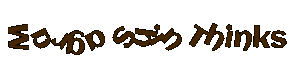# The Book of Wisdom – Episode 5 – Why Should We Change?

• 19

HELP
NL1

• 10 + 10 + 10 = 30, schoenen zijn 10

5 + 5 + 10 = 20, vrouw is 5

4 + 4 + 5 = 13, de map is 4

Map (4) + vrouw (5) + schoenen (10) = 19

• Answer

Scroll+human+boot=?

4+5+10=19

Ign lll

ts4.idFinal Turnamen 2020 dimulai 17.11.2020

• The answer is = 19

server : tx10.arabics.travian.com

Player : time

• 33

• 33

Madnessity

ts20.travian.com Codex Victoria X2

Post was edited 1 time, last by Ridder Huma: email removed ().

• 1 pair of boots = 10 points

1 woman = 5 points

1 scroll = 4 points

1 scroll + 1 woman ( she wears boots and holds a scroll) + 1 pair of boots = 4 + 5 + 10 + 4 + 10 = 33 is the answer

• ooh.. This is my real true answer. Delete the others.

True Answer is..

Scroll+(human+(boot+scroll))+boot=?

4+(5+(10+4))+10= 33

33 is the answer.

Ign lll

ts4.idFinal Turnamen 2020 dimulai 17.11.2020

• Answer is = 19

ID : Alwerfalli

Server: tx10.arabics

• Fidht Com 3

Boots. : 10

Woman : 5

Map (Last Item) : 4

Total : Map + Woman + Boots = 19• Do i solve the problem and respond here?